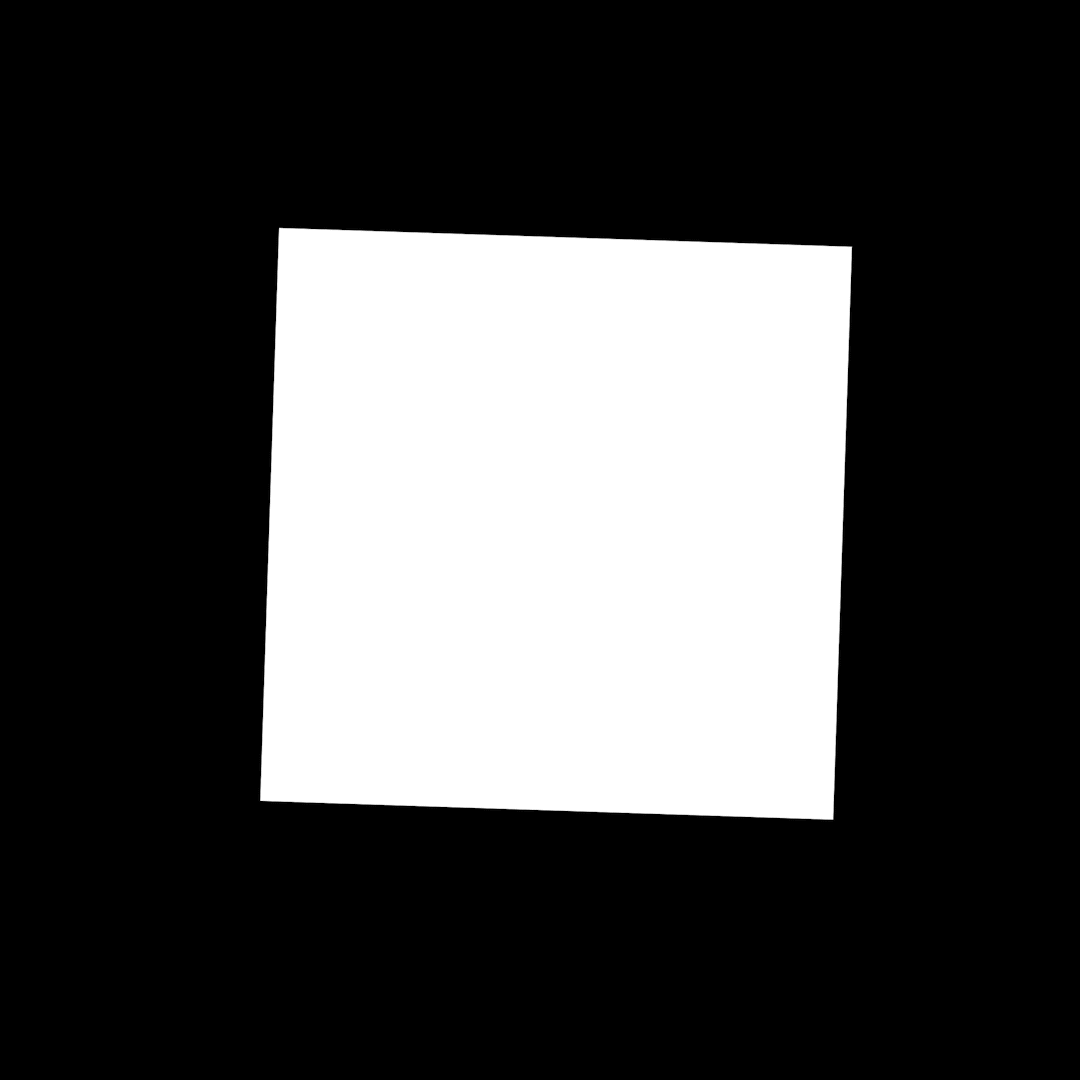Expression Library

# Bobbing Object (On Undulating Surface)

Author: Dan Ebberts

This After Effects expression will create motion that simulates the movement of an object that is floating on waves. The author of this expression, Dan Ebberts called this effect “undulations” and wrote that it “simulates an undulating surface”, but note that it will not warp your layer to look like a flag or other surface with waves moving across it. That effect can be achieved using the wave warp effect.

## Example

Here’s an example of this effect in action:## Code Sample

Hover over the code and click the copy button.

```				```

xAmp = 25; //height of undulations (pixels)
xFreq = .3; //undulations per second
xSpeed = 150; //speed of wave (pixels per second)

wl = xSpeed/xFreq; //wavelength (pixels)
phaseOffset = ((position%wl)/wl)*2*Math.PI;
y = xAmp*Math.sin(2*Math.PI*xFreq*time + phaseOffset);
value + [0,y]